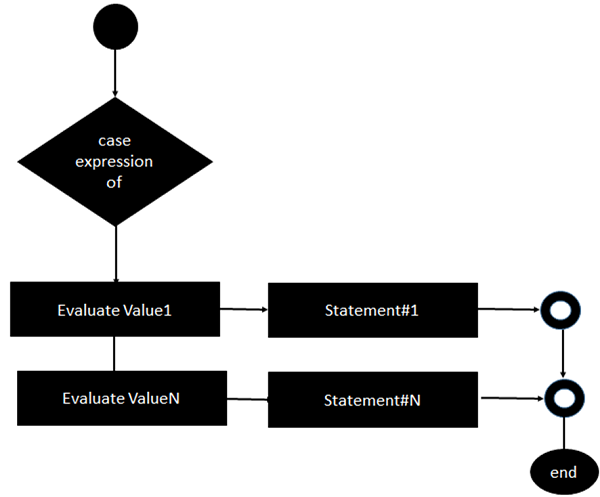# Erlang - Case Statements

Erlang offers the case statement, which can be used to execute expressions based on the output of the case statement.

The general form of this statement is −

## Syntax

```case expression of
value1 -> statement#1;
value2 -> statement#2;
valueN -> statement#N
end.
```

The general working of this statement is as follows −

• The expression to be evaluated is placed in the case statement. This generally will evaluate to a value, which is used in the subsequent statements.

• Each value is evaluated against that which is passed by the case expression. Depending on which value holds true, that subsequent statement will be executed.

The following diagram shows the flow of the case statement.The following program is an example of the case statement in Erlang −

## Example

```-module(helloworld).
-export([start/0]).

start() ->
A = 5,
case A of
5 -> io:fwrite("The value of A is 5");
6 -> io:fwrite("The value of A is 6")
end.
```

The output of the above code will be −

## Output

```The value of A is 5.
```
erlang_decision_making.htm